# An aqueous solution of a nonvolatile solute has a boiling point of 102.45 degrees Celsius. Knowing...

An aqueous solution of a nonvolatile solute has a boiling point of 102.45 degrees Celsius. Knowing that for water, Kfp = 1.86 degrees Celsius m^-1 and Kbp= 0.51 degrees Celsius m^-1. What is the molality of the solution? and what is the freezing point of the solution? Please show work.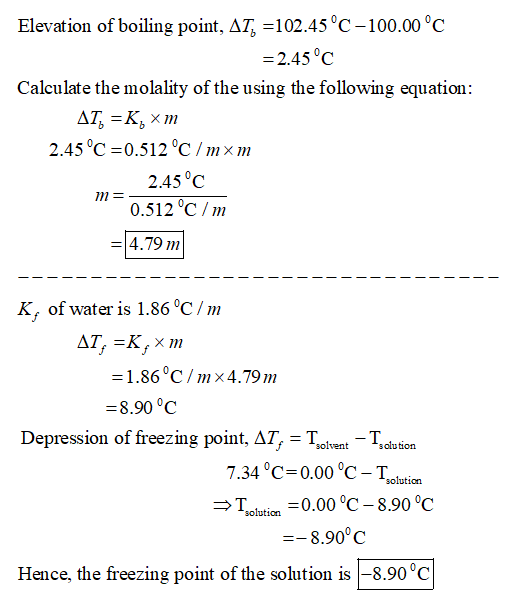#### Earn Coin

Coins can be redeemed for fabulous gifts.

Similar Homework Help Questions
• ### An aqueous solution has a normal boiling point of 103 c. What is the freezing point...

An aqueous solution has a normal boiling point of 103 c. What is the freezing point of his solution? For Water Kb= 0.51 C/m and Kf= 1.86 C/, I want the answer with datels please!!

• ### If the boiling point of a solution was 101.8 degrees Celsius and the boiling point of...

If the boiling point of a solution was 101.8 degrees Celsius and the boiling point of pure water under the same conditions was 99.7 degrees Celsius, what is the molality of the solution?

• ### A Review Constants Periodic Table The changes in boiling point (AT) or freezing point (AT) in...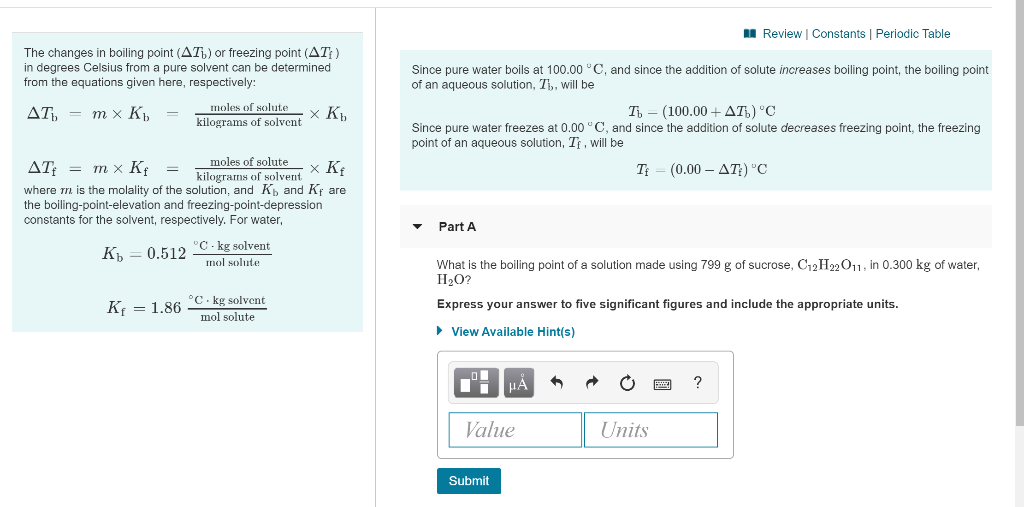A Review Constants Periodic Table The changes in boiling point (AT) or freezing point (AT) in degrees Celsius from a pure solvent can be determined from the equations given here, respectively: AT) = m x K = moles of solute XK K. kilograms of solvent Since pure water boils at 100.00 °C, and since the addition of solute increases boiling point, the boiling point of an aqueous solution, Th, will be T - (100.00+AT) 'C Since pure water freezes at...

• ### Review Constants Periodic Table The changes in boiling point (AT) or freezing point (AT) in degrees...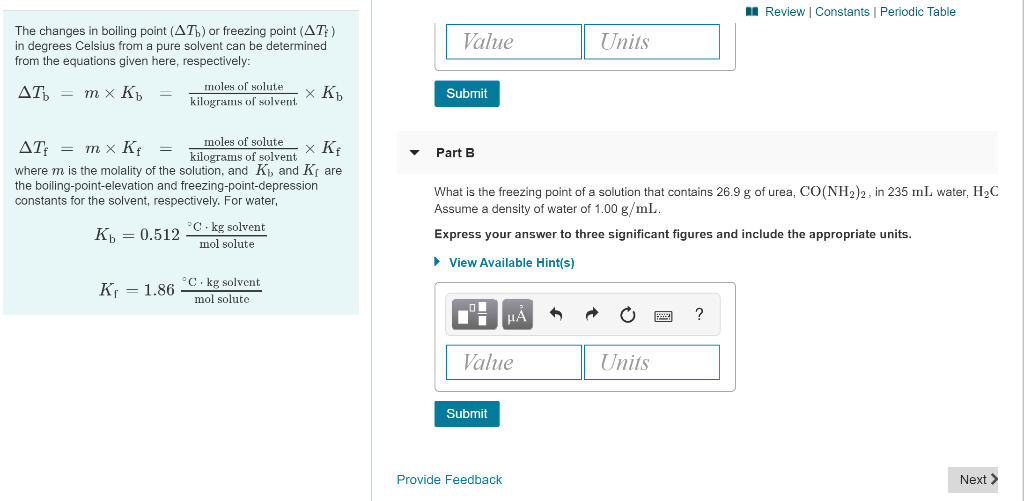Review Constants Periodic Table The changes in boiling point (AT) or freezing point (AT) in degrees Celsius from a pure solvent can be determined from the equations given here, respectively: Value Units moles of solute AT = mx Kb = 7 Submit kilograms of solvent XRb moles of solutex Kf Part B AT: = mx Kf = kilograms of solvent where m is the molality of the solution, and K and K the boiling-point-elevation and freezing-point-depression constants for the solvent,...

• ### A solution of a nonvolatile solute in water has a boiling point of 381.2 .

A solution of a nonvolatile solute in water has a boiling point of 381.2 K .Calculate the vapor pressure of water above this solution at 338 K . The vapor pressure of pure water at this temperature is 0.2467 atm .

• ### What is the normal boiling point of an aqueous solution that has a freezing point of...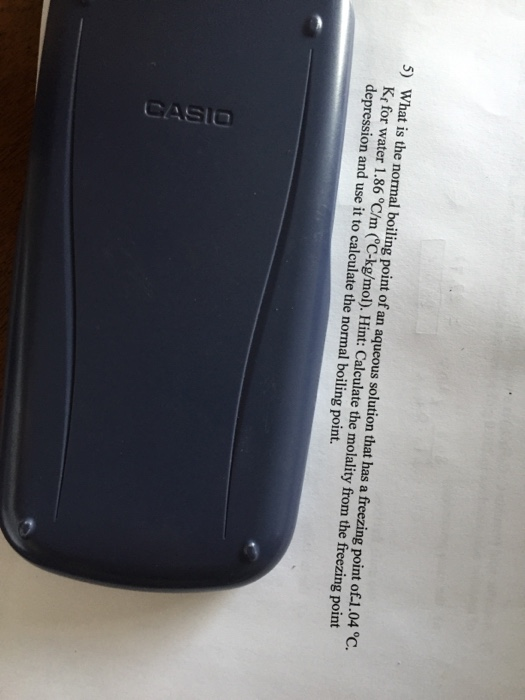What is the normal boiling point of an aqueous solution that has a freezing point of 1.04 degree C. K_f for water 1.86 degree C/m.

• ### a Calculate the freezing point and the boiling point of each of the following aqueous solutions....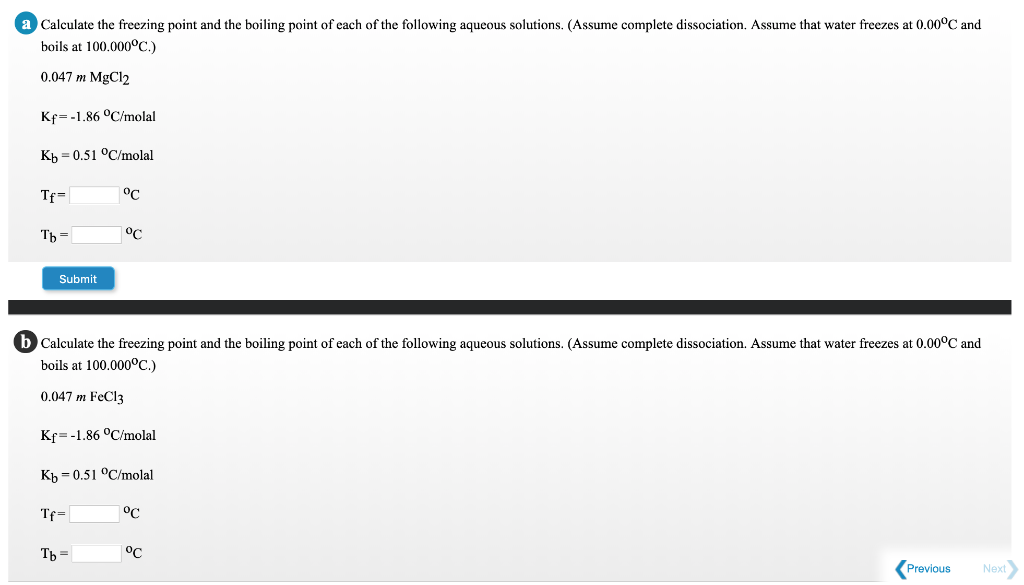a Calculate the freezing point and the boiling point of each of the following aqueous solutions. (Assume complete dissociation. Assume that water freezes at 0.00°C and boils at 100.000ºC.) 0.047 m MgCl2 Kr=-1.86 °C/molal Kb =0.51 °C/molal Tf= °C Tb = °C Submit b Calculate the freezing point and the boiling point of each of the following aqueous solutions. (Assume complete dissociation. Assume that water freezes at 0.00°C and boils at 100.000°C.) 0.047 m FeCl3 Kf=-1.86 °C/molal Kb =0.51 °C/molal...

• ### 14. What is the vapor pressure in mmHg of a solution of a solution that contains...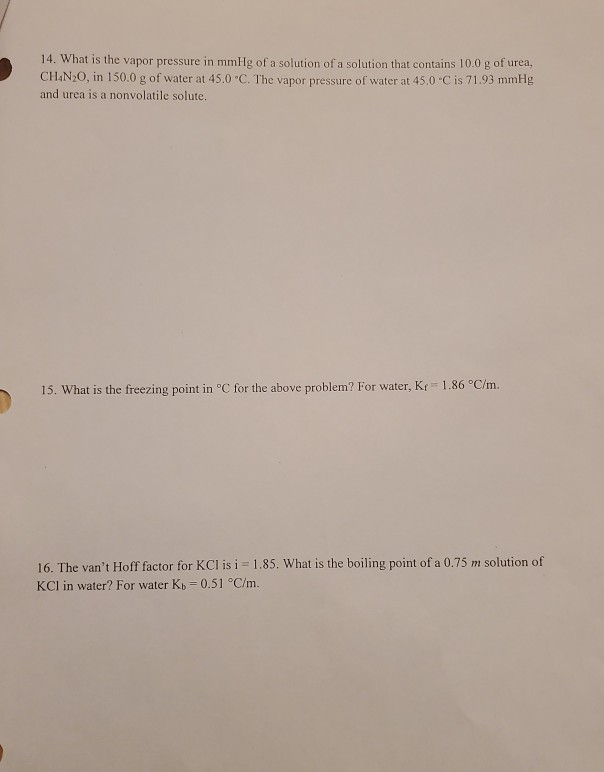14. What is the vapor pressure in mmHg of a solution of a solution that contains 10.0 g of urea, CHAN20, in 150.0 g of water at 45.0 "C. The vapor pressure of water at 45.0°C is 71.93 mmHg and urea is a nonvolatile solute. 15. What is the freezing point in °C for the above problem? For water, Kr-1.86 °C/m. 16. The van't Hoff factor for KClisi = 1.85. What is the boiling point of a 0.75 m solution...

• ### A pure solvent freezes at 6.35 degrees Celsius and a pure solute freezes at 832.0 degrees...

A pure solvent freezes at 6.35 degrees Celsius and a pure solute freezes at 832.0 degrees Celsius. The molar mass of the solvent is 96.4 g/mol and the molar mass of the solute is 78.5 g/mol. The freezing point depression constant for the solvent is 6.33 degrees/m. A solution is made of 1.012 g of the solute and 15.280 g of the solvent, determine its freezing point in degrees Celsius.

• ### Choose the aqueous solution that has the highest boiling point. These are all solutions of nonvolatile...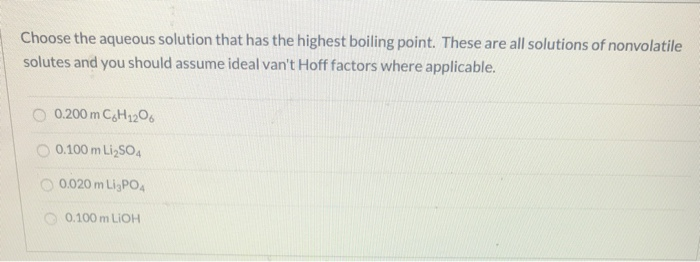Choose the aqueous solution that has the highest boiling point. These are all solutions of nonvolatile solutes and you should assume ideal van't Hoff factors where applicable. O 0.200 m C6H1206 0.100 m Li2SO4 0.020 m LigPO 0.100 m LIOH